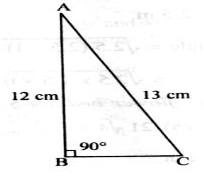# Test: Heron's Formula- 2

## 25 Questions MCQ Test Mathematics (Maths) Class 9 | Test: Heron's Formula- 2

Description
This mock test of Test: Heron's Formula- 2 for Class 9 helps you for every Class 9 entrance exam. This contains 25 Multiple Choice Questions for Class 9 Test: Heron's Formula- 2 (mcq) to study with solutions a complete question bank. The solved questions answers in this Test: Heron's Formula- 2 quiz give you a good mix of easy questions and tough questions. Class 9 students definitely take this Test: Heron's Formula- 2 exercise for a better result in the exam. You can find other Test: Heron's Formula- 2 extra questions, long questions & short questions for Class 9 on EduRev as well by searching above.
QUESTION: 1

### Find the length of each side of an equilateral triangle having area of 9 root 3 cm square

Solution:

Given area of equilateral triangle = 9√3 cm²

Also,

Area of the equilateral triangle = √3/4 × (side)²

So,

√3/4 × (side)² = 9√3

side² = (9√3 × 4)/√3

side² = 36

side = √36

side = 6cm

QUESTION: 2

Solution:

A = bh

500 = b x 20

b =  500/20

b = 25 cm

QUESTION: 3

### The area of an isosceles right angled triangle of equal side 30 cm, is given as

Solution:
QUESTION: 4

If side of a scalene △ is doubled then area would be increased by

Solution:
QUESTION: 5

An isosceles right triangle has area 8 cm2. The length of its hypotenuse is :

Solution:
QUESTION: 6

If the area of an equilateral triangle is 36√3 cm2, then the perimeter of the triangle is

Solution:
QUESTION: 7

Given the product of diagonals of a rhombus ABCD is 2500 cm2, its area is

Solution:
QUESTION: 8

The area and length of one diagonal of a rhombus are given as 200 cm2 and 10 cm respectively. The length of other diagonal is

Solution:
QUESTION: 9

In each side of a △ is halved then its perimeter will be decreased by

Solution:
QUESTION: 10

Area of the given triangle isSolution:
QUESTION: 11

The edges of a triangular board are 6 cm, 8 cm and 10 cm. The cost of painting it at the rate of 70 paise per cm2 is

Solution:
QUESTION: 12

The area of a right angled triangle if the radius of its circumcircle is 3 cm and altitude drawn to the hypotenuse is 2 cm.

Solution:
QUESTION: 13

The area of one triangular part of a rhombus ABCD is given as 125 cm2. The area of rhombus ABCD is

Solution:
QUESTION: 14

Length of perpendicular drawn on smallest side of scalene triangle is

Solution:
QUESTION: 15

The sides of a triangle are in ratio 3 : 4 : 5. If the perimeter of the triangle is 84 cm, then area of the triangle is :

Solution:
QUESTION: 16

The perimeter of a rhombus is 20 cm. If one of its diagonals is 6 cm, then its area is

Solution:
QUESTION: 17

Area of an isosceles triangle ABC with AB = a = AC and BC = b is

Solution:
QUESTION: 18

Each side of an equilateral triangle measures 10 cm. Then the area of the triangle is

Solution:
QUESTION: 19

Length of perpendicular drawn on longest side of a scale △ is

Solution:
QUESTION: 20

Each side of an equilateral triangle is 2x cm. If x√3 = √48, then area of the triangle is :

Solution:
QUESTION: 21

The area of a rhombus of 96 cm2. If one of its diagonals is 16 cm, then the length of its side is

Solution:
QUESTION: 22

The area of quadrilateral ABCD whose diagonals are perpendicular and of lengths 12 cm, 8 cm is

Solution:
QUESTION: 23

The area of a parallelogram whose base is 32 m and the corresponding altitude is 6 m is

Solution:

area of parallelogram = base x height

=32 x 6 = 192 m2

QUESTION: 24

Semiperimeter of scalene triangle of side k, 2k and 3k is

Solution:
QUESTION: 25

The sides of a triangle are x, y and z. If x + y = 7 m, y + z = 9 m, and z + x = 8 m, then area of the triangle is :

Solution:

x + y = 7....(1)
y + z = 9....(2)
z + x = 8....(3)
So, 2(x+y+z) = 24
x+y+z = 12
Now, y + z = 9  then x = 3
z + x = 8 then y = 4
x + y = 7 then z = 5
We can see that, this becomes a right angled triangle.
Hence, Area of triangle = 1/2 * base * perpendicular
= 1/2 * 4 * 3
= 6 sq unit.